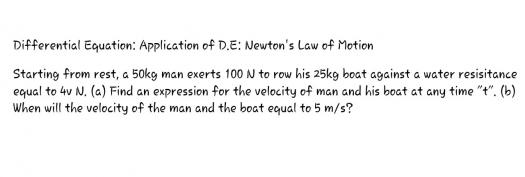# Differential Equation: Application of D.E: Newton's Law of Motion

2 posts / 0 new
Ednalyn DG CarpioDifferential Equation: Application of D.E: Newton's Law of Motionfitzmerl duron#1.

To get the expression of the velocity of the man-boat system, start by getting the net force...

Net force = $100$ N - $60$ N = $40$ N

Getting the acceleration of the system using Newton's second law of motion:

$$Force = mass \times acceleration$$ $$F = ma$$
Then...

$$a = \frac{F}{m}$$ $$a = \frac{40 \space newtons}{25\space kg + 50 \space kg}$$ $$a = 0.53 \space \frac{m}{s^2}$$

Using the kinematic equation $v_f = v_i+at$, where $v_f$ is the final velocity, $v_i$ is the initial velocity, $a$ is the acceleration and $t$ is time

So...
$$v_f = v_i+at$$

Get the acceleration $a$:
$$a = \frac{v_f-v_i}{t}$$ $$0.53 \space \frac{m}{s^2} = \frac{v_f-0 \space \frac{m}{s}}{t}$$

Then express the final velocity $v_f$ of the boat-man system in terms of time:

$\color {green}{v_f = 0.53t}$

#2

The time needed for the boat-man system to reach $5 \space \frac{m}{s}$ would be...

$$v_f = 0.53t$$ $$5 \space \frac{m}{s} = 0.53t$$ $$\color{green}{t = 9.4 \space seconds}$$

Alternate solutions are highly encouraged.....

• Mathematics inside the configured delimiters is rendered by MathJax. The default math delimiters are $$...$$ and $...$ for displayed mathematics, and $...$ and $...$ for in-line mathematics.